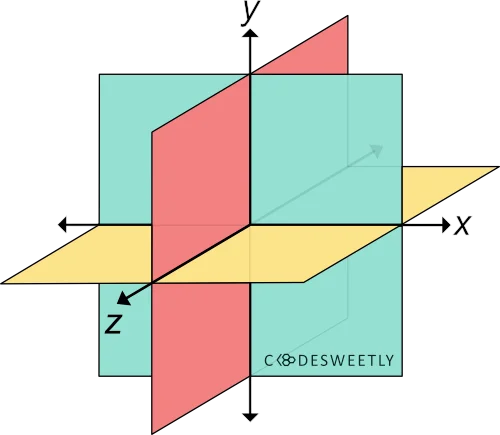# CSS rotateX() Function – How to Rotate Elements around X-axis

rotateX() transforms an element by rotating it three-dimensionally around the X-axis.A three-dimensional Cartesian coordinate system showing the X-, Y-, and Z-axis

## Syntax of the CSS rotateX() Function​

rotateX() accepts a single argument. Here is the syntax:

element {  transform: rotateX(angle);}

Note the following:

• The rotateX(angle) function is equivalent to rotate3d(1, 0, 0, angle).
• The angle argument specifies the element's angle of rotation.
• angle can be in degree, gradian, radian, or turn.
• An angle argument consists of a number followed by the unit you wish to use—for instance, 45deg.

## Examples of the CSS rotateX() Function​

Below are some examples of how the CSS rotateX() function works.

### How to do a zero-degree rotation around the X-axis​

img {  transform: rotateX(0deg);  width: 80%;}

Try Editing It

The snippet above used the rotateX() function to specify a zero-degree (0⁰) rotation for the image around the X-axis.

### How to do a 70-degree rotation around the X-axis​

img {  transform: rotateX(70deg);  width: 80%;}

Try Editing It

The snippet above used the rotateX() function to specify a seventy-degree (70⁰) rotation for the image around the X-axis.

## CSS rotateX() Function vs. rotate Property: What's the Difference?​

CSS rotateX() functions and CSS rotate property provide two similar ways to specify rotation transformations.

The main differences between the two rotation techniques are as follows:

• The CSS rotate property allows rotating an element without using the CSS transform property.
• The CSS rotate property's syntax is shorter than its function alternative.
• The CSS rotate property saves you from remembering the specific order to position the transform functions.
• Browsers calculate the transform functions' matrix in the order you assigned them to the CSS transform property—from left to right.
• Browsers calculate the transform properties' matrix in the following transformation matrix order:
1. translate
2. rotate
3. scale

Here's an example:

Use CSS rotate property to do a 70-degree rotation around the X-axis:

img {  rotate: x 70deg; /* Equal to a transform: rotateX(70deg) property */  width: 80%;}

Try Editing It

The snippet above used the rotate property to specify a seventy-degree (70⁰) rotation for the image around the X-axis.

note

A none value tells browsers not to rotate the selected element.

## Overview​

This article discussed what a CSS rotateX() function is. We also used examples to see how it works.